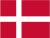### Dish Cloth 65

Cast on 60 stitches and knit 6 rows, and then pattern. Remember to start and end all rows with 2 k. When the cloth has the desired length you knit 6 rows and bind off.

Pattern:

1.row: knit
2.row: 2 p, 3 k, 3 p, 3 k, 3 p
3.row: 2 k, 3 p, 1 k, 1 p, 1 k, 1 p, 1 k, 3 p, 1 k
4.row: 3 k, 1 p, 2 k, 1 p, 2 k, 1 p, 3 k, 1 p
5.row: 1 k, 2 p, 1 k, 3 p, 1 k, 3 p, 1 k, 2 p
6.row: 1 k, 1 p, 3 k, 3 p, 3 k, 1 p, 1 k, 1 p
7.row: 2 k, 3 p, 5 k, 3 p, 1 k
8.row: purl
9.row: as row 7
10.row: as row 6
11.row: as row 5
12.row: as row 4
13.row: as row 3
14.row: as row 2

 X X X X X X 14 X X X X X X X X 13 X X X X X X X X X X 12 X X X X X X X X X X 11 X X X X X X X X 10 X X X X X X 9 8 X X X X X X 7 X X X X X X X X 6 X X X X X X X X X X 5 X X X X X X X X X X 4 X X X X X X X X 3 X X X X X X 2 1
You have to remember that all uneven rows start in the right side of the diagram and X=purl and ▢= knit. All equal rows start in the left side of the diagram and X=knit and ▢=purl.Danish